## 2.5 创建一个涟漪效果#

public static float Ripple(float x, float z, float t)
{
float d = Mathf.Sqrt(x * x + z * z);
float y = d;
return y;
}


static GraphFunction[] functions =
{
SineFunction,
MultiSineFunction,
Sine2DFunction,
MultiSine2DFunction,
Ripple,
};

public enum GraphFunctionName
{
Sine,
MultiSine,
Sine2D,
MultiSine2D,
Ripple,
}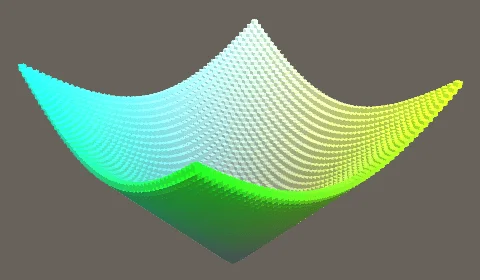float d = Mathf.Sqrt(x * x + z * z);
float y = Mathf.Sin(pi * d);
return y;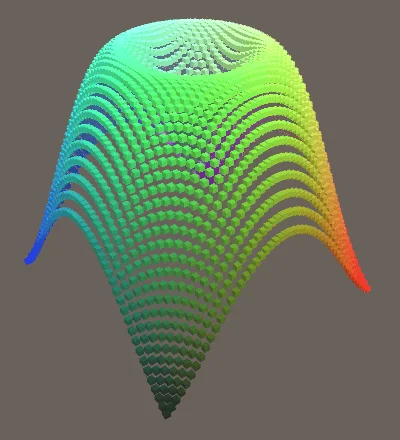float y = Mathf.Sin(4f * pi * d);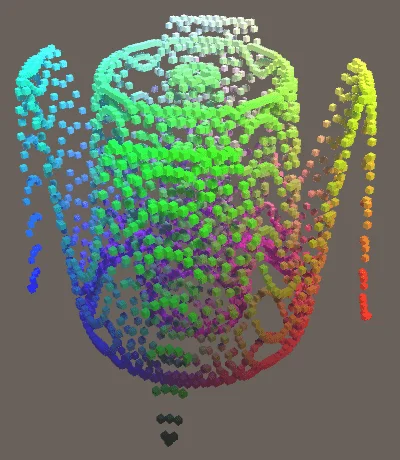public static float Ripple(float x, float z, float t)
{
float d = Mathf.Sqrt(x * x + z * z);
float y = Mathf.Sin(4f * pi * d);
y /= 1f + 10f * d;
return y;
}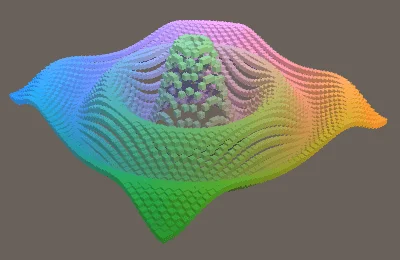public static float Ripple(float x, float z, float t)
{
float d = Mathf.Sqrt(x * x + z * z);
float y = Mathf.Sin(pi * (4f * d - t));
y /= 1f + 10f * d;
return y;
}


## 3.1 三维函数#

public delegate Vector3 GraphFunction(float u, float v, float t);


public static Vector3 SineFunction(float x, float z, float t)
{
float y = Mathf.Sin(pi * (x + t));
Vector3 p = new Vector3(x, y, z);
return p;
}

public static Vector3 MultiSineFunction(float x, float z, float t)
{
float y = Mathf.Sin(pi * (x + t));
y += Mathf.Sin(2f * pi * (x + 2f * t)) / 2f;
y *= 2f / 3f;
Vector3 p = new Vector3(x, y, z);
return p;
}

public static Vector3 Sine2DFunction(float x, float z, float t)
{
float y = Mathf.Sin(pi * (x + t));
y += Mathf.Sin(pi * (z + t));
y *= 0.5f;
Vector3 p = new Vector3(x, y, z);
return p;
}

public static Vector3 MultiSine2DFunction(float x, float z, float t)
{
float y = 4f * Mathf.Sin(pi * x + z + t * 0.5f);
y += Mathf.Sin(pi * (x + t));
y += Mathf.Sin(2f * pi * (z + 2f * t)) * 0.5f;
y *= 1f / 5.5f;
Vector3 p = new Vector3(x, y, z);
return p;
}

public static Vector3 Ripple(float x, float z, float t)
{
float d = Mathf.Sqrt(x * x + z * z);
float y = Mathf.Sin(pi * (4f * d - t));
y /= 1f + 10f * d;
Vector3 p = new Vector3(x, y, z);
return p;
}


void Update()
{
float t = Time.time;
GraphFunction f = functions[(int)function];

float step = 2f / resolution;
for(int i = 0, z = 0; z < resolution; ++z)
{
float v = (z + 0.5f) * step - 1f;
for(int x = 0; x < resolution; ++x, ++i)
{
float u = (x + 0.5f) * step - 1f;
points[i].localPosition = f(u, v, t);
}
}
}


void Awake()
{
float step = 2f / resolution;
Vector3 scale = Vector3.one * step;
points = new Transform[resolution * resolution];

for(int i = 0; i < points.Length; ++i)
{
Transform point = Instantiate(pointPrefab);
point.localScale = scale;
point.SetParent(transform, false);
points[i] = point;
}
}


## 3.2 创建一个圆柱体#

public static Vector3 Cylinder (float u, float v, float t)
{
Vector3 p;
p.x = 0f;
p.y = 0f;
p.z = 0f;
return p;
}


public static Vector3 Cylinder (float u, float v, float t)
{
Vector3 p;
p.x = Mathf.Sin(pi * u);
p.y = 0f;
p.z = Mathf.Cos(pi * u);
return p;
}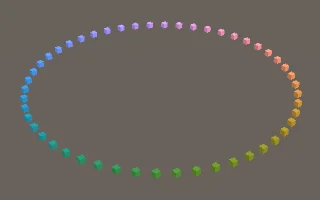public static Vector3 Cylinder (float u, float v, float t)
{
Vector3 p;
p.x = Mathf.Sin(pi * u);
p.y = v;
p.z = Mathf.Cos(pi * u);
return p;
}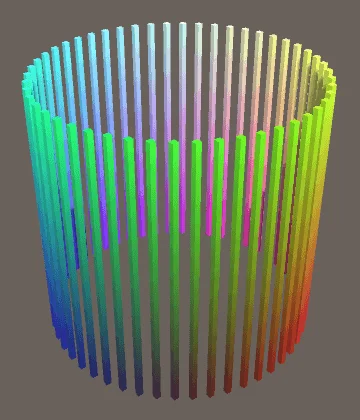public static Vector3 Cylinder (float u, float v, float t)
{
Vector3 p;
float r = 1f;
p.x = r * Mathf.Sin(pi * u);
p.y = v;
p.z = r * Mathf.Cos(pi * u);
return p;
}


float r = 1f + Mathf.Sin(6f * pi * u) * 0.2f;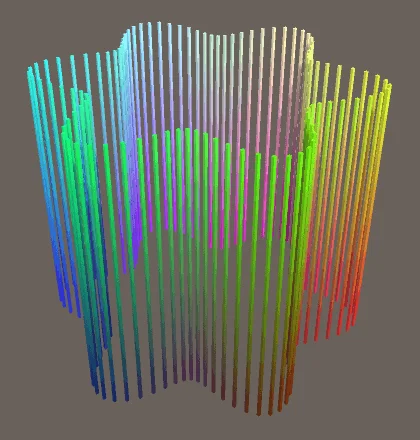float r = 1f + Mathf.Sin(2f * pi * v) * 0.2f;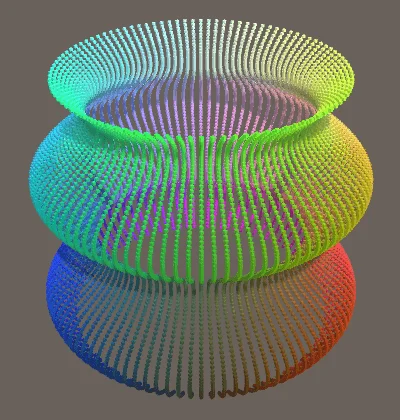float r = 0.8f + Mathf.Sin(pi * (6f * u + 2f * v + t)) * 0.2f;


## 3.3 创建一个球体#

public static Vector3 Sphere (float u, float v, float t) {
Vector3 p;
float r = Mathf.Cos(pi * 0.5f * v);
p.x = r * Mathf.Sin(pi * u);
p.y = v;
p.z = r * Mathf.Cos(pi * u);
return p;
}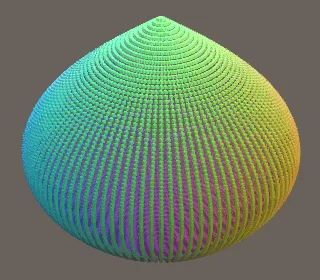p.y = Mathf.Sin(pi * 0.5f * v);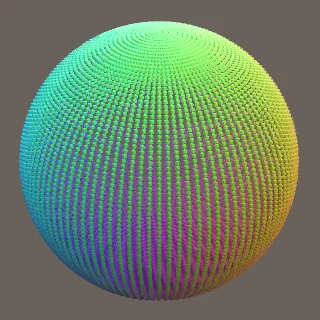float r = 0.8f + Mathf.Sin(pi * (6f * u + t)) * 0.1f;
r += Mathf.Sin(pi * (4f * v + t)) * 0.1f;
float s = r * Mathf.Cos(pi * 0.5f * v);
p.x = s * Mathf.Sin(pi * u);
p.y = r * Mathf.Sin(pi * 0.5f * v);
p.z = s * Mathf.Cos(pi * u);


## 3.4 创建一个环面#

public static Vector3 Torus (float u, float v, float t) {
Vector3 p;
float s = Mathf.Cos(pi * 0.5f * v);
p.x = s * Mathf.Sin(pi * u);
p.y = Mathf.Sin(pi * 0.5f * v);
p.z = s * Mathf.Cos(pi * u);
return p;
}


float s = Mathf.Cos(pi * 0.5f * v) + 0.5f;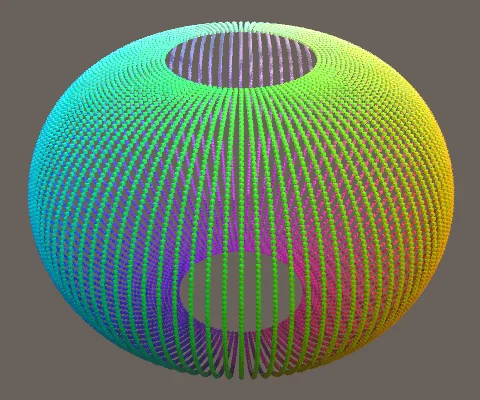float s = Mathf.Cos(pi * v) + 0.5f;
p.x = s * Mathf.Sin(pi * u);
p.y = Mathf.Sin(pi * v);
p.z = s * Mathf.Cos(pi * u);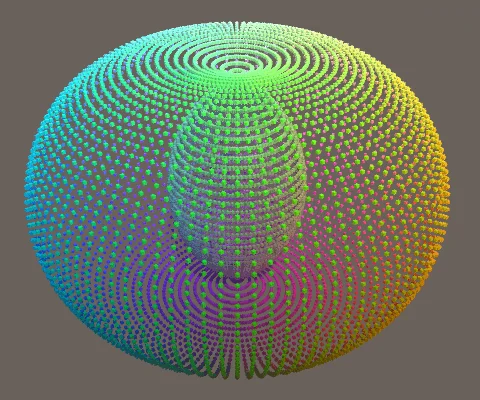float r1 = 1f;
float s = Mathf.Cos(pi * v) + r1;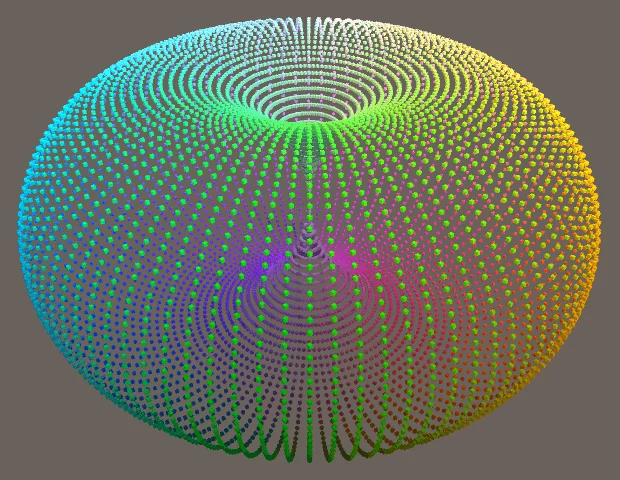float r1 = 1f;
float r2 = 0.5f;
float s = r2 * Mathf.Cos(pi * v) + r1;
p.x = s * Mathf.Sin(pi * u);
p.y = r2 * Mathf.Sin(pi * v);
p.z = s * Mathf.Cos(pi * u);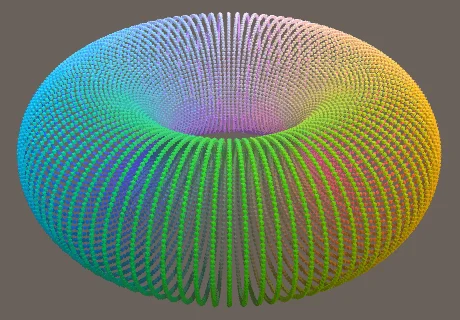float r1 = 0.65f + Mathf.Sin(pi * (6f * u + t)) * 0.1f;
float r2 = 0.2f + Mathf.Sin(pi * (4f * v + t)) * 0.05f;